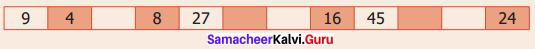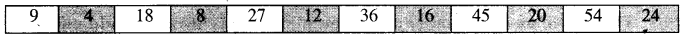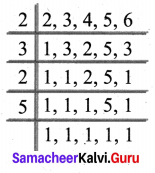## Tamilnadu Samacheer Kalvi 6th Maths Solutions Term 2 Chapter 1 Numbers Intext Questions

Try These (Textbook Page No. 1)

Question 1.
(i) Observe and complete:
1 + 3 = ?
5 + 11 = ?
21 + 47 = ?
___ + ____ = ?
From this observation, we conclude that “the sum of any two odd numbers is always an _____”
(ii) Observe and complete:
5 × 3 = ?
7 × 9 = ?
11 × 13 = ?
_____ × ____ = ?
From this observation, we conclude that “the product of any two odd numbers is always an _____”
Justify the following statements with appropriate examples:
(iii) The sum of an odd number and an even number is always an odd number.
(iv) The product of an odd and an even number is always an even number.
(v) The product of only three odd numbers is always an odd number.
Solution:
(i) 1 + 3 = 4
5 + 11 = 16
21 + 47 = 68
An odd number + another odd number = An Even number
From this observation, we conclude that the sum of any two odd numbers is always an even number.
(ii) 5 × 3 = 15
7 × 9 = 63
11 × 13 = 143
An odd number × Another odd number = An odd number
From this observation, we conclude that “the product of any two odd numbers is always an odd number.”
(iii) Take the odd number 5 and the even number 10
Their sum = 5 + 10 = 15, which is odd.
∴ Sum of an odd number and an even number is always an odd number.
(iv) Take the odd number 5 and the even number 10.
Their product = 5 × 10 = 50, which is even
Thus the product of an odd and an even number is always an even number.
(v) Consider 7 × 5 × 3
We know that the product of any two odd numbers is an odd number
7 × 5 = 35, odd number.
Also we have 35 × 3 = 105
∴ 7 × 5 × 3 = 105, an odd number.
So the product of three odd numbers is always an odd number.Try These (Textbook Page No. 3)

Question 1.
(i) Say True or False
(a) The smallest odd natural number is 1.
(b) 2 is the smallest even whole number.
(c) 12345 + 5063 is an odd number.
(d) Every number is a factor of itself.
(e) A number which is a multiple of 6 is also a multiple of 2 and 3.
(ii) Is 7, a factor of 27?
(iii) Is 12, a factor or a multiple of 12?
(iv) Is 30, a factor or a multiple of 10?
(v) Which of the following numbers has 3 as a factor?
(a) 8
(b) 10
(c) 12
(d) 14
(vi) The factors of 24 are 1, 2, 3, __, 6, ___, 12 and 24. Find the missing ones.
(vii) Look at the following numbers carefully and find the missing multiples.Solution:
(i) (a) True
(b) False
(c) False
(d) True
(e) True
(ii) No, 7 is not a factor of 27. Because 7 does not divide 27 exactly
(iii) 12 is both a factor and a multiple of 12
(iv) 30 is a multiple of 10
(v) (a) Factors of 8 are 1, 2, 4, 8
(b) Factors of 10 are 1, 2, 5, 10
(c) Factors of 2 are 1, 2, 3, 4, 6, 12
(d) Factors of 14 are 1, 2, 7, 14
∴ The number 12 has 3 as a factor
(vi) Factors of 24 are 1, 2, 3, 4, 6, 8, 12, 24.
Missing Factors 4, 8.
(vii)Try These (Textbook Page No. 6)

Question 1.
Express 68 and 128 as the sum of two consecutive primes.
Solution:
68 = 31 + 37
128 = 61 + 67

Question 2.
Express 79 and 104 as the sum of any three odd primes.
Solution:
79 = 37 + 31 + 11
79 = 41 + 31 + 7
79 = 61 + 11 + 7
79 = 59 + 13 + 7
79 = 53 + 19 + 7 and so on.
104 cannot be expressed as the sum of three odd primes.
Because we know that “ the sum of any two odd numbers is an even number”.
Also the sum of an odd and even number is always an odd number.
104 = 61 + 41 + 2
104 = 97 + 5 + 2
104 = 89 + 13 + 2 and so on.Try These (Textbook Page No. 8)

Question 1.
Are the leap years divisible by 2?
Solution:
Leap years are divisible by 4.
Leap years are divisible by 2.

Question 2.
Is the first 4 digit number divisible by 3?
Solution:
The first four-digit number is 1000.
Sum of the digits is 1 + 0 + 0 + 0 = 1, not divisible by 3.
1000 is not divisible by 3.

Question 3.
Is your date of birth (DDMMYYYY) divisible by 3?
Solution:
Date of birth 25.05.2007
Sum of digits = 2 + 5 + 0 + 5 + 2 + 0 + 0 + 7 = 21
Again 2 + 1 = 3, divisible by 3.
My date of birth is divisible by 3.

Question 4.
Identify the numbers in the sequence 2000, 2006, 2010, 2015, 2019, 2025 that are divisible by both 2 and 5.
Solution:
We know that a number is divisible by both 2 and 5 if it is divisible by 10. 2000 and 2010 are divisible by 10.

Question 5.
Check whether the sum of 5 consecutive numbers is divisible by 5.
Solution:
Take the first five consecutive natural numbers 1, 2, 3, 4 and 5.
Their sum 1 + 2 + 3 + 4 + 5 = 15, divisible by 5.
Also, 2 + 3 + 4 + 5 + 6 = 20, divisible by 5.
3 + 4 + 5 + 6 + 7 = 25, divisible by 5.
Generally, the sum of 5 consecutive natural numbers is divisible by 5.

Try These (Textbook Page No. 19)

A small boy went to a town to sell a basket of wood apples. On the way, some robbers grabbed the fruits from him and ate them! The small boy went to the King and complained. The King asked him, “How many wood apples did you bring?”. The boy replied, “Your Majesty! I didn’t know, but I knew that if you divided my fruits into groups of 2, one fruit would be left in the basket”. He continued saying that if the fruits were divided into groups of 3, 4, 5 and 6, the fruits left in the basket would be 2, 3, 4 and 5 respectively. Also, if the fruits were divided into groups of 7, no fruit would be there in the basket. Can you find the number of fruits, the small boy had initially?
(This problem is taken from the famous Mathematics problems collection book in Tamil called “Kanakkathikaram” under the heading of “Wood Apple Problem”)
Solution:
The total number of fruits, when divided by 2, 3, 4, 5 and 6, leaves the remainders 1, 2, 3, 4 and 5 respectively.
Here (2 – 1) = (3 – 2) = (4 – 3) = (5 – 4) = (6 – 5) = 1.
∴ The required no. of fruits will be LCM (2, 3, 4, 5, 6) – 1
L CM (2, 3, 4, 5, 6) = 2 × 3 × 2 × 5 = 60Now take the multiples of 60 and subtract 1 from it.
Also checking the conditions, multiplies of 60 are 60, 120, 180, …..
The multiple -1
59, 119, 179, ……
The required number = 119
∴ The total number of fruits = 119.